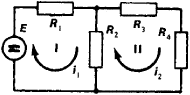# Mesh Current Method

The following article is from The Great Soviet Encyclopedia (1979). It might be outdated or ideologically biased.

## Mesh Current Method

(also mesh analysis or loop analysis), a method of computation of electric circuits, in which the currents in meshes formed by an arbitrary division of the circuit are taken as unknowns. For example, the electric circuit shown in Figure 1 is divided into meshes I and II and clockwise mesh currents are labeled as i1 and i2.Figure 1. Diagram of an electric circuit with two meshes (I and II)

According to Kirchhoffs second law, the set of equations that can be written for the mesh currents is

R1i1 + R2i1R2i2 = E (for mesh I)

R2i1 + R2i2 + R3i2 + R4i2 = 0 (for mesh II)

The values of currents i1 and i2 may be found by solving these equations simultaneously; the branch currents, which do not depend on the arbitrary selection of meshes, are equal to the algebraic sum of the mesh currents. If the electric circuit is divided into n meshes, the number of mesh currents and the number of equations will also be equal to n. The resulting set of n linear equations in n unknowns can be solved by standard methods of linear algebra. The mesh current method may be used for computation of both DC and AC circuits.

### REFERENCE

Bessonov, L. A. Teoreticheskie Osnovy elektrotekhniki, 5th ed. Moscow, 1967.

V. V. BOGOMAZOV